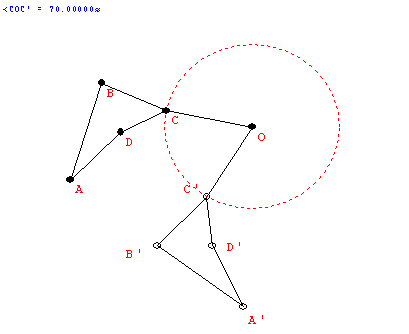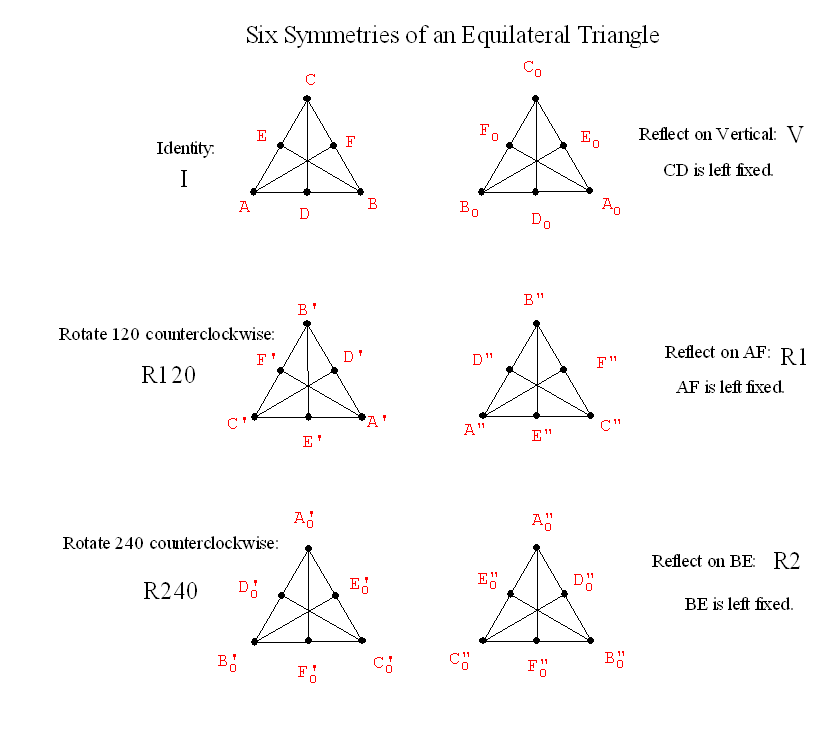February 10

Review from last class:

• In general: The individual angle for a regular polygon with n sides is (n-2)*180/n degrees.
This can be expressed in other ways using algebra:
(n-2)*180/n = [180 n - 360] / n = 180 - 360/n.
• An on-line tool for making tilings of the plane
• Regular and semiregular Tilings of the plane.
• A tiling is a regular tiling if(i)it has a single tile shape that is a regular polygon and (ii) the vertices and edges of the tiles coincide (no overlapping edges)
• A tiling is a semi-regular tiling if (ii) each tile shape is a regular polygon, (ii) the vertices and edges of the tiles coincide (no overlapping edges) and (iii) every vertex has the same polygon types arranged around it.
• Naming tilings (Math Forum)
• The numbers represent the number of sides in the poygons.
• The order indcates the order in which the poygons are arranged about a vertex.
• Local considerations about a vertex. The sum of the angles must equal 360 degrees.
• 4.1  Ex. 3. How many tiles around single vertex: 3,4,5,6,7,8?...
• How can there be 6? Only one way: 3-3-3-3-3-3   (6*60= 360)
• Why not 7? If 7 or more tiles aroung a single vertex the sum of the angles must exceed 360 degrees.
• 4.1  Ex. 4. Can there be 4 different tiles around a single vertex? Not if they are all different since 60+90+108+120 = 378 >360.
• 4.1  Ex. 5. If there are 4 tiles around a vertex then ....At least two of the tiles must have the same number of sides.

• Arithmetic for vertices.

• Example: Three regular polygons about a vertex with n , k, and p sides.

(180 - 360/n) + (180 - 360/k) + (180 - 360/p) = 360

3*180 -360( 1/n+1/k+1/p)= 2*180

1*180 = 360( 1/n+1/k+1/p)

SO....
180/360 = 1/n + 1/k + 1/p  or
1/n + 1/k + 1/p=1/2

So, for example, n=3, k=4 and p= 5 is not possible since

1/3+1/4+1/5 >1/2.

Number of polygons
around a vertex
Equation for angle sum = 360 Equivalent Arithmetic equation Solutions to the arithmetic equations.
3: n , k, p 180 - 360/n+180 - 360/k+180 - 360/p = 360 1/n+1/k+1/p =1/2  6 6 6 5 5 10 4 5 20 4 6 12 4 8 8 3 7 42 3 8 24 3 9 18 3 10 15 3 12 12

4:  n, k, p, z 180 - 360/n+180 - 360/k+180 - 360/p 180 - 360/z = 360 1/n+1/k+1/p +1/z =2/2 =1  4 4 4 4 3 3 4 12 3 3 6 6 3 4 4 6

5:  n, k, p, z, w 180 - 360/n+180 - 360/k+180 - 360/p+180 - 360/z+180 - 360/w = 360 1/n+1/k+1/p +1/z+1/w =3/2  3 3 3 3 6 3 3 3 4 4

• Local consideration about a polygon:
• Examples: If there is an equilateral triangle involved with 2 other polygons, then the other two ploygons must have the same number of sides. Because: if there were two different polygons around the triangle, then one vertex would not have all three polygons sharing that vertex. (such as 3-10-15)
• Similar considerations can eliminate tilings using a single pentagon and two other distinct tiles. (such as 4-5-20).

• Semiregular Tilings: global results!
Look at the results using wingeometry.
• Student lesson (Math Forum) a place for further explorations on-line.
• Show FAPP video on Patterns
• as review of tilings so far and
• Introduces Penrose Tiles  K&M section 4.3 as source for possible protfolio/project ideas.
Duality Activity
Why is this called "duality"?
Back and forth in the construction
We place a point in each  region(polygon) .
Every vertex of the old figure is surrounded by a region( polygon) in the new figure.
"Having two forms"...   point.... region

Other dualities?

Symmetry Ideas
• Reflective symmetry: BI LATERAL SYmMETRY
• Folding line: "axis of symmetry"
• The "flip."
• The "mirror."
•• R(P) = P'  : A Transformation. Before: P .... After : P'

• If P is on the line (axis), then F(P)=P.
"P remains fixed by the reflection."

• If P is not on the axis,
then the line PP' is perpendicular to the axis and
if Q is the point of intersection of PP' with the axis then m(PQ) = m(P'Q).

• Definition: We say F has a reflective symmetry wrt a line l if  there is a reflection  R about the line l where  R(P) is still an element of F for every P in F....i.e.. R (F) = F.
• Examples of reflective symmetry:

• Rotational Symmetry.
• Center of rotation. "rotational pole" (usually O) and angle/direction of rotation.
• The "spin."
•• R(P) = P' : A transformation
• If O is the center then F(O) = O.
• If the angle is 360 then F(P) = P for all P.... called the identity transformation.
• If  the angle is between 0 and 360 then only the center remains fixed.
• For any point P the angle POP'  is the same.
• Examples of rotational symmetry.

•  Now what about finding all the reflective and rotational symmetries of a single figure?

•• Why are there only six?
• What about combining transformations to give new symmetries: